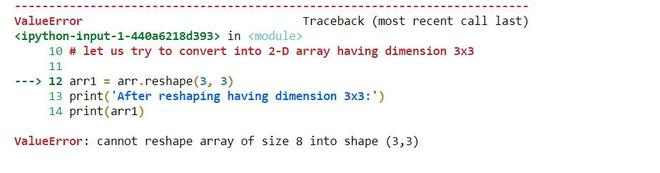Related Articles
Convert a 1D array to a 2D Numpy array
• Last Updated : 13 Jan, 2021

Numpy is a Python package that consists of multidimensional array objects and a collection of operations or routines to perform various operations on the array and processing of the array. This package consists of a function called numpy.reshape which is used to convert a 1-D array into a 2-D array of required dimensions (n x m).  This function gives a new required shape without changing the data of the 1-D array.

Syntax: numpy.reshape(array, new_shape, order)

Parameters:

•  array: is the given 1-D array that will be given a new shape or converted into 2-D array
• new_shape: is the required shape or 2-D array having int or tuple of int
• order: ‘C’ for C style, ‘F’ for Fortran style, ‘A’ if data is in Fortran style then Fortran like order else C style.

Examples 1:

## Python3

 `import` `numpy as np``# 1-D array having elements [1 2 3 4 5 6 7 8]``arr ``=` `np.array([``1``, ``2``, ``3``, ``4``, ``5``, ``6``, ``7``, ``8``])`` ` `# Print the 1-D array``print` `(``'Before reshaping:'``)``print` `(arr)``print` `(``'\n'``)`` ` `# Now we can convert this 1-D array into 2-D in two ways`` ` `# 1. having dimension 4 x 2``arr1 ``=` `arr.reshape(``4``, ``2``)``print` `(``'After reshaping having dimension 4x2:'``)``print` `(arr1)``print` `(``'\n'``)`` ` `# 2. having dimension 2 x 4``arr2 ``=` `arr.reshape(``2``, ``4``)``print` `(``'After reshaping having dimension 2x4:'``)``print` `(arr2)``print` `(``'\n'``)`

Output:

```Before reshaping:
[1 2 3 4 5 6 7 8]
After reshaping having dimension 4x2:
[[1 2]
[3 4]
[5 6]
[7 8]]
After reshaping having dimension 2x4:
[[1 2 3 4]
[5 6 7 8]]```

Example 2: Let us see an important observation that whether we can reshape a 1-D array into any 2-D array.

## Python3

 `import` `numpy as np``# 1-D array having elements [1 2 3 4 5 6 7 8]``arr ``=` `np.array([``1``, ``2``, ``3``, ``4``, ``5``, ``6``, ``7``, ``8``])`` ` `# Print the 1-D array``print``(``'Before reshaping:'``)``print``(arr)``print``(``'\n'``)`` ` `# let us try to convert into 2-D array having dimension 3x3``arr1 ``=` `arr.reshape(``3``, ``3``)``print``(``'After reshaping having dimension 3x3:'``)``print``(arr1)``print``(``'\n'``)`

Output:This concludes that the number of elements should be equal to the product of dimension i.e. 3×3=9 but total elements = 8;

Example 3: Another example is that we can use the reshape method without specifying the exact number for one of the dimensions. Just pass -1 as the value and NumPy will calculate the number.

## Python3

 `import` `numpy as np``# 1-D array having elements [1 2 3 4 5 6 7 8]``arr ``=` `np.array([``1``, ``2``, ``3``, ``4``, ``5``, ``6``, ``7``, ``8``])`` ` `# Print the 1-D array``print``(``'Before reshaping:'``)``print``(arr)``print``(``'\n'``)`` ` ` ` `arr1 ``=` `arr.reshape(``2``, ``2``, ``-``1``)``print``(``'After reshaping:'``)``print``(arr1)``print``(``'\n'``)`

Output:

```Before reshaping:
[1 2 3 4 5 6 7 8]
After reshaping:
[[[1 2]
[3 4]]
[[5 6]
[7 8]]]```

Attention geek! Strengthen your foundations with the Python Programming Foundation Course and learn the basics.

To begin with, your interview preparations Enhance your Data Structures concepts with the Python DS Course.

My Personal Notes arrow_drop_up